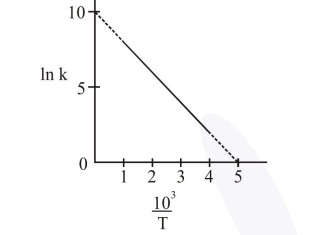# The rate constant k of a reaction is measured at different temperatures (T), and the data are

Question:

The rate constant (k) of a reaction is measured at different temperatures (T), and the data are plotted in the given figure. The activation energy of the reaction in $\mathrm{kJ} \mathrm{mol}^{-1}$ is :

( $R$ is gas constant)1. $2 \mathrm{R}$

2. $R$

3. $1 / R$

4. $2 / \mathrm{R}$

Correct Option: 1

Solution:

Slope $=-\frac{E_{a}}{R}$

$-\frac{10}{5}=-\frac{E_{a}}{R}$

$\mathrm{E}_{\mathrm{a}}=2 \mathrm{R}$